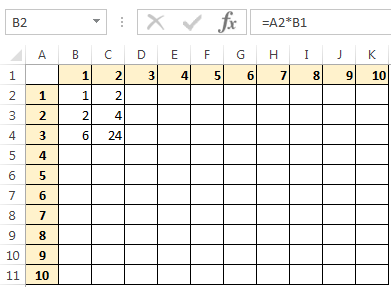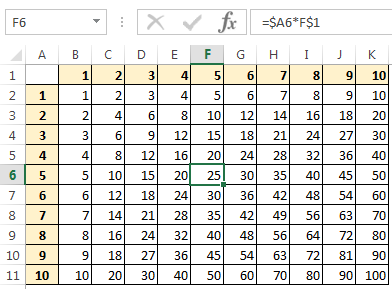# The Challenge

In the last blog post I set a challenge for you.

Create a table similar to the one below, in cell B2, create a formula that can be copied to all other cells in the table.  The result of this will be a multiplication table.# The Solution

The solution in theory is very simple, multiply the numbers in row 1 by the numbers in column A.  The formula in B2 would then be =A2*B1

which works, but if you copy this formula to a few more cells you can see it goes wrong.3 times 2 is not 24 (even I know that!).

Our formula is a relative formula.

If you copy it 2 columns to the right of the original, the column letter increases by 2.  If you copy the formula down 2 rows the row number increases by 2.

So, the formula in C4 is =B4*C3 which is 6*4=24.

We will need to use a mixed reference formula.

We want to multiply row 1 every time by column A, so we will make this part of the formula absolute, this is done by adding a dollar sign \$ before the column letter or row number so that they don’t change when the formula is copied to other cells.

The solution to the problem is =\$A2*B\$1 and we can copy that formula across rest of the cells.Now when you copy the formula, it will always reference column A and row 1, but the other half of each cell name will still be relative.

Did you get it yourself?

[grwebform url=”http://app.getresponse.com/view_webform.js?wid=4307503&u=CucD” css=”on”/]